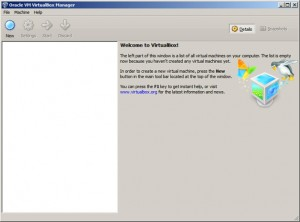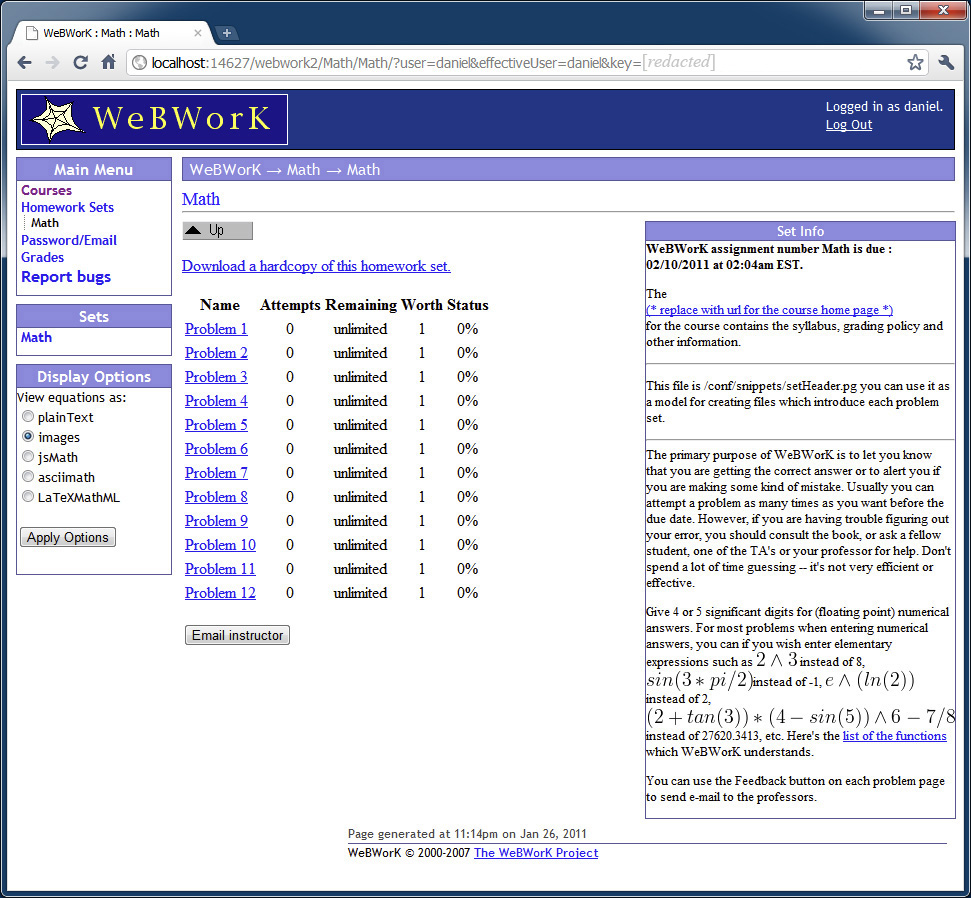Categories

## Predicting Y When the Dependent Variable is a Transformation of Y

I am going to try to start posting more frequently. This post covers topics that I’ve been thinking about lately, including model estimation with ordinary least squares (OLS) and forecasting when OLS is used to fit a statistical model with a dependent variable that is a transformation of some variable we wish to forecast.

Suppose we run a regression with the following specification:$Y=\beta_{0}+\beta_{1}X_{1}+\beta_{2}X_{2}+\ldots+\beta_{n}X_{n}+\varepsilon$

Let’s assume that the error term is distributed normally, and let’s use OLS to solve for the coefficients in the model. Using a superscript to denote the m observations in the dataset, our m-by-n+1 design matrix$\mathbf{X}$ is$\left[\begin{array}{ccccc} 1 & X_{1}^{(1)} & X_{2}^{(1)} & \cdots & X_{n}^{(1)}\\ 1 & X_{1}^{(2)} & X_{2}^{(2)} & \cdots & X_{n}^{(2)}\\ \vdots & \vdots & \vdots & \ddots & \vdots\\ 1 & X_{1}^{(m)} & X_{2}^{(m)} & \cdots & X_{n}^{(m)}\end{array}\right]$

If the coefficient vector is labeled$\overrightarrow{\beta}$ and the vector containing the Y variable’s values is labeled$\overrightarrow{y}$, then the OLS estimation for the coefficients can be calculated by solving$\mathbf{X}^{\top}\mathbf{X}\overrightarrow{\beta}=\mathbf{X}^{\top}\overrightarrow{y}$ for$\overrightarrow{\beta}$.$\overrightarrow{\beta}=\left(\mathbf{X}^{\top}\mathbf{X}\right)^{-1}\mathbf{X}^{\top}\overrightarrow{y}$

Now we have a set of coefficients that we can use to predict values of Y when we receive additional observations that have values for our independent variables X1, X2, …, Xn, and no observed values of Y. Everything is fine.

Categories

## Probability Distributions Reference Table

Table Description

I have uploaded a reference table that I created with common probability distributions. Although the same formulas and similar descriptions can be obtained on Wikipedia, I find it useful to have the information contained on one table. The table does not have charts that plot probability density or probability mass functions (PDFs and PMFs) or cumulative distribution functions (CDFs). These charts can be found on Wikipedia or plotted with graphing software using the equations on the table. I find it helpful to look at PDF and CDF plots when learning about the distributions. There might be some closed form CDFs, moment generating functions, or other equations that I omitted from the table. Also, some distributions have various formulations. For example, the four distributions defined below are all very similar, and I imagine that each might be referred to as a negative binomial, although certain specifications are more conventional than others.

1. A distribution of the number of failures in a sequence of Bernoulli trials, before a specified number of successes.
2. A distribution of the number of successes in a sequence of Bernoulli trials, before a specified number of failures.
3. A distribution of the number of successes and failures in a sequence of Bernoulli trials, until a specified number of successes.
4. A distribution of the number of successes and failures in a sequence of Bernoulli trials, until a specified number of failures.

In cases where there are multiple ways to define a distribution, I either used the definition that I prefer, or gave multiple formulations of the distribution. I am including a link to both a PDF of the table and an Excel file that has the table and a VBA macro that centers images across a column or multiple columns. Also included is a link to the MathML markup file that was used to generate the equations on the table. Excel’s equation editor was giving me problems, and I did not want to type$\LaTeX$ code manually, so I searched for a what you see is what you get (WYSIWYG) equation editor on the internet. I ended up finding an open source project, Formulator MathML Editor (http://code.google.com/p/formulator-mathml/), which I think is great. After getting started, I also found a$\LaTeX$ editor, LyX (http://www.lyx.org/), which probably would have worked just as well, with the advantage that I’m more familiar with$\LaTeX$ than MathML. I have spotted and corrected a few errors that I made when creating the table (e.g., I originally had the continuous uniform distribution’s variance equal to$\frac{1}{12}\left(b+a\right)^{2}$, which was corrected to$\frac{1}{12}\left(b-a\right)^{2}$), but there still may be some errors. Please let me know if you find any.

Categories

## Installing Your Own Personal WeBWork Server, Part III

Part IPart II | Part III | Part IV

This series of posts covers the installation of WeBWork. This post, Part III of the series, has instructions for installing Ubuntu and WeBWork within VirtualBox.

Creating a New Virtual Machine

The first step requires the configuration of a new virtual machine in VirtualBox. This new virtual machine will be used to install a copy of Ubuntu with WeBWork. To configure a new virtual machine, you must click New from the VirtualBox Manager. The images below show the progression of steps and my selected settings when configuring a new virtual machine. Please customize these settings according to your preferences and system. For example, if you are using a 32-bit system, please make sure to select Ubuntu (32 bit) when prompted, as opposed to the 64-bit option that I selected.Categories

## Installing Your Own Personal WeBWork Server, Part II

Part I | Part II | Part III | Part IV

This series of posts covers the installation of WeBWork. This post, Part II of the series, has instructions for installing VirtualBox. Although I am using Windows 7, the instructions should apply to other operating systems that VirtualBox can be installed on.

Why Install VirtualBox?

WeBWork is designed to be installed alongside a web server on a Unix based computer. VirtualBox is a software program that allows you to install additional operating systems within the operating system you are currently running. Another option would be to install WeBWork on a separate computer that is dedicated to web hosting. While this option may be appealing to colleges, it did not seem practical for the purpose of installing my own personal version of WeBWork. Additionally, the option presented here has a lower cost, as VirtualBox is available for free. For additional details on VirtualBox, please check out its homepage and/or the corresponding Wikipedia page.

Categories

## Installing Your Own Personal WeBWork Server, Part I

Part IPart II | Part III | Part IVThis series of posts will give step-by-step instructions on installing your own WeBWork server. WeBWork is an open source, freely available homework system. It is used by many colleges, and is often installed on a college network. While its installation may sound complicated, as it is typically installed on a Linux computer running Apache with a SQL database, these instructions will make it very simple to install your own personal copy of WeBWork on Windows and Mac computers. Knowledge of Linux, Apache, and SQL will not be necessary. Installing WeBWork will give you access to over 20,000 freely available problems that span topics ranging from discrete mathematics to multivariable calculus.